Function Repository Resource:

# StupidSort

Just like Sort, but works much more stupidly

Contributed by: Tang Anke
 ResourceFunction["StupidSort"][list] inefficiently sorts the elements of list into canonical order.

## Details and Options

The time complexity is about O(n3logn).
ResourceFunction["StupidSort"] algorithmically replaces list with the rule {x___,a_,b_,y___}/;a>b{x,b,a,y} until no match can be found.

## Examples

### Basic Examples (2)

Create an array of 10 random values:

 In:=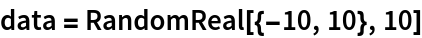Out=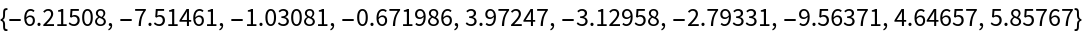Stupidly sort the random array:

 In:=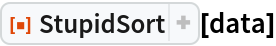Out=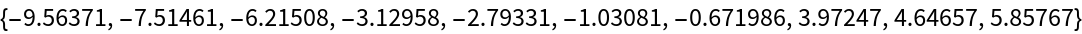### Properties and Relations (5)

Create an array:

 In:=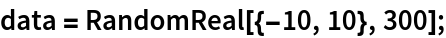StupidSort takes several seconds to sort the data:

 In:=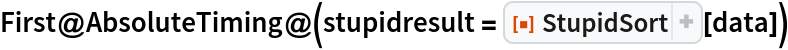Out=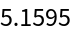In:=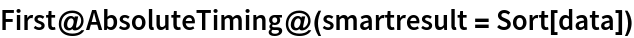Out=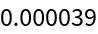Sort is much less stupid:

 In:=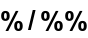Out=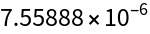Even so, both methods give the same result:

 In:=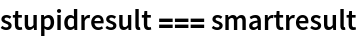Out=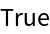Tang anke

## Version History

• 1.0.0 – 26 March 2020